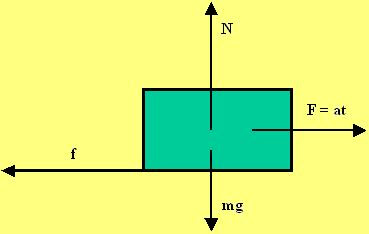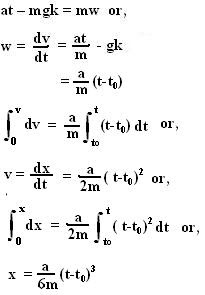## Sunday, November 25, 2007

### Irodov Problem 1.103The force diagram is depicted in the Figure beside. Initially the force will be too weak to overcome the friction force f offered by the surface. As time goes by the force at will exceed the maximum possible value of f that the surface has to offer namely Nk. At this time the mass will start to accelerate. Let us call this time when the body starts to move as t0.

Forces in the vertical direction
There are two forces acting in the vertical direction, i) the force of gravity mg and ii) the Normal reaction from the surface N. There is no component of acceleration for the body in the vertical direction. Thus we have,

N - mg = 0 (1)

Forces in the horizontal direction
There are two forces acting on the body in the horizontal direction, i) the force F=at and ii) the force of friction f. Let the acceleration of the body be w. Thus we have,

at - f = mw (2)

At the point when the body begins to move f = Nk, F=at0 and w=0. Thus, from (1) and (2) we have,After time
t0 the mass begins to accelerate. During this time the friction force f is fixed at mgk . From (1) and (2) we have,